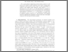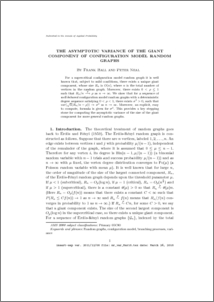The asymptotic variance of the giant component of configuration model random graphs

Ball, Frank and Neal, Peter John (2017) The asymptotic variance of the giant component of configuration model random graphs. Annals of Applied Probability, 27 (2). pp. 1057-1092. ISSN 1050-5164Preview
PDF (mr_var_Mar16)
mr_var_Mar16.pdf - Accepted Version

Abstract

For a supercritical configuration model random graph it is well known that, subject to mild conditions, there exists a unique giant component, whose size $R_n$ is $O (n)$, where $n$ is the total number of vertices in the random graph. Moreover, there exists $0 < \rho \leq 1$ such that $R_n/n \convp \rho$ as $\nr$. We show that for a sequence of {\it well-behaved} configuration model random graphs with a deterministic degree sequence satisfying $0 < \rho < 1$, there exists $\sigma^2 > 0$, such that $var (\sqrt{n} (R_n/n -\rho)) \rightarrow \sigma^2$ as $\nr$. Moreover, an explicit, easy to compute, formula is given for $\sigma^2$. This provides a key stepping stone for computing the asymptotic variance of the size of the giant component for more general random graphs.

Item Type:
Journal Article
Journal or Publication Title:
Annals of Applied Probability
Uncontrolled Keywords:
/dk/atira/pure/subjectarea/asjc/1800/1804
Subjects:
Departments:
ID Code:
80084
Deposited By:
Deposited On:
16 Jun 2016 12:30
Refereed?:
Yes
Published?:
Published